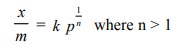Home | | Engineering Chemistry | Adsorption Isotherms

## Chapter: Engineering Chemistry: Surface Chemistry and Catalysis

The adsorption isotherm of a gas which is adsorbed on a solid.

The extent of adsorption is measured in terms of the quantity  x/m  where, x is the mass of the gas (adsorbate) adsorbed at equilibrium on mass m of the adsorbent. x/m  is the mass of the adsorbate adsorbed per unit mass of the adsorbent. The graph showing variation in x/m with pressure(p) at a constant temperature is called adsorption isotherm. Let us see the variation in extent of adsorption in case of gases and of solutes from their solutions.

The adsorption isotherm of a gas which is adsorbed on a solid is shown in Fig. 17.5. It shows that the extent of adsorption of a gas on a solid increases with the increase in the pressure of the gas, p at three different constant temperatures. The curves also show that the extent of adsorption, decreases at a fixed pressure as the temperature is increased (see the dotted line).Freundlich gave an empirical mathematical relationship between the extent of adsorption (x/m) and the equilibrium pressure (p) of the gas as :In this relation k is a constant at a given temperature and depends upon the nature of the adsorbate and adsorbent. The value of n changes with pressure. It is 1 at low pressures and increases with pressure. The relationship is valid at a constant temperature. Therefore, it is called Freundlich Adsorption Isotherm. On taking logarithm of the above equation, we getThis is an equation of a straight line and a plot of log x/m against log p should be a straight line with slope 1/n as depicted in Fig. 17.6. In actual practice, a straight line is obtained provided the data at very low and very high pressures is neglected.Study Material, Lecturing Notes, Assignment, Reference, Wiki description explanation, brief detail
Engineering Chemistry: Surface Chemistry and Catalysis : Adsorption Isotherms |